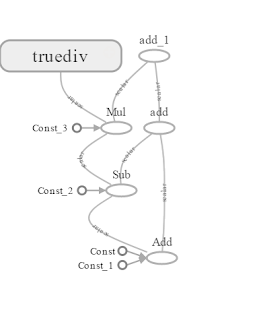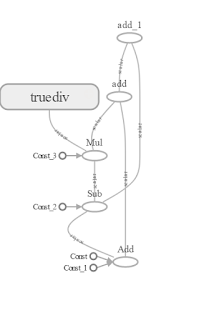### Arithmetic Operations in Tensorflow

In this article, we will learn about basic arithmetic operators supported by the Tensorflow. We will also perform these operations on the tensors and create a computational graph which can be viewed with the help of the Tensorboard.

To get started, let's create two tensors of type constants.
``````# Create two nodes
node1 = tf.constant(35)  # dtype is int32
node2 = tf.constant(76)  # dtype is int32``````
``````# Add node1 and node2
Ok now, lets learn how to subtract the two nodes. For this, I'm going to take our previous added result node_add as the first node and node3 as the second node.
``````#  Create node3
node3 = tf.constant(11)  # dtype is int32
node_subtract = tf.subtract(node_add, node3)  # dtype is int32``````
Similarly, we use node_subtract, node4 for multiplication and node_multiply, node5 for division once we created the node4, node5.
``````# Create node4
node4 = tf.constant(10)  # dtype is int32
# Create node5
node5 = tf.constant(100)  # dtype is int32

# Multiply node_subtract and node4
node_multiply = tf.multiply(node_subtract, node4)  # dtype is int32
# Divide node_multiply and node5
node_divide = tf.divide(node_multiply, node5)  # dtype is float32``````
This is the basic implementation of arithmetic operations in TensorFlow and for more arithmetic operations you can check the TensorFlow site.

Sometimes, we may want to perform arithmetic operations on more than two nodes. In that case, we have to perform an arithmetic operation on first two nodes then on the result, the next consecutive node and so on.

Let's say we want to add three nodes or four nodes or five nodes, If we use the official formula, we end with some thing like this
``````# Adding three nodes
``````# Output node or Final node
output = node_add + node_subtract + node_multiply``````
Both give same results and you can use any one or both in your code. This extends to other operators too (eg: - or tf.subtract, * or tf.multiply).

You can also set your output to be
``output = node_add + node_subtract + node_multiply + node_divide``
If you use this, you'll get a Type Error saying that Tensor conversion requested dtype int32 for Tensor with dtype float64. To get rid of this, you need to convert the node_divide which is of type Float32 to int32.

Now, let's start a session to print the output value and create the computational graph.
``````# Start the session
with tf.Session() as sess:
# Output value
output_value = sess.run(output)
print('Session: Output value: ', output_value)
# Create a summary
summary = tf.Summary(value=[
tf.Summary.Value(tag="summary_basic_math", simple_value=output_value)
])
# Create the event file inside logs directory
writer = tf.summary.FileWriter("logs/", sess.graph)
# Add the summary to the writer
The computational graph was created in the logs folder and is waiting to be visualized in the Tensorboard. Below is the entire code and is also available as a gist.
``````'''
Class performs arithmetic operations on multiple tensors and
shows the Computational graph with the help of Tensorboard.

Uses constants for arithmetic operations
'''
import tensorflow as tf

def main():
'''
Main Method
'''
# Create tensors
node1 = tf.constant(35)
node2 = tf.constant(76)
node3 = tf.constant(11)
node4 = tf.constant(10)
node5 = tf.constant(100)

print('Node 1: ', node1)  # Tensor("Const:0", shape=(), dtype=int32)
print('Node 2: ', node2)  # Tensor("Const_1:0", shape=(), dtype=int32)
print('Node 3: ', node3)  # Tensor("Const_2:0", shape=(), dtype=int32)
print('Node 4: ', node4)  # Tensor("Const_3:0", shape=(), dtype=int32)
print('Node 5: ', node5)  # Tensor("Const_4:0", shape=(), dtype=int32)

print('Node Subtract: ', node_subtract)  # Tensor("Sub:0", shape=(), dtype=int32)

# Multiply node_subtract and node4
node_multiply = tf.multiply(node_subtract, node4)
print('Node Multiply: ', node_multiply)  # Tensor("Mul:0", shape=(), dtype=int32)

# Divide node_multiply and node5
node_divide = tf.divide(node_multiply, node5)
print('Node Divide: ', node_divide)  # Tensor("truediv:0", shape=(), dtype=float64)

# Output node or Final node
output = node_add + node_subtract + node_multiply
print('Ouptut Node: ', output)  # Tensor("add_1:0", shape=(), dtype=int32)

# Start the session
with tf.Session() as sess:
# Output value
output_value = sess.run(output)
print('Session: Output value: ', output_value)  # Output value:  1211
# Create a summary
summary = tf.Summary(value=[
tf.Summary.Value(tag="summary_basic_math", simple_value=output_value)
])
# Create the event file inside logs directory
writer = tf.summary.FileWriter("logs/", sess.graph)
# Add the summary to the writer

if __name__ == "__main__":
'''
Starting point
'''
# This file is being run directly
main()``````
Here are different ways to add multiple tensors.
``````output1 = node_add + node_subtract + node_multiply
output2 = node_subtract + node_multiply + node_add
output3 = node_multiply + node_add + node_subtract``````
All the outputs (output1, output2, output3) give you the same result but their computational graph will be different.

For output1 node_add is added to node_subtract first and the result will be added to node_multiply. For output3 node_multiply is added to node_add first and the result is added to node_subtract. You can see the effect on the computational graph in the below figures.Fig: output1 computational graphFig: output3 computational graph